﻿ 基于微波链路的天气雷达降水场校准方法
 快速检索 中文标题 英文标题 作者中文名 作者英文名 单位中文名 单位英文名 中文关键词 英文关键词 中文摘要 英文摘要 基金项目 全文
 气象2023, Vol. 49Issue (2): 157-169.  DOI: 10.7519/j.issn.1000-0526.2022.061801### 引用本文 [复制中英文]

[复制中文]
ZHANG Peng, LIU Xichuan, ZHOU Zeming, et al, 2023. Calibration of Weather Radar Rainfall Field Based on Microwave Links[J]. Meteorological Monthly, 49(2): 157-169. DOI: 10.7519/j.issn.1000-0526.2022.061801.
[复制英文]

### 文章历史

2021年12月21日收稿
2022年8月14日收修定稿

1. 陆军工程大学教研保障中心，南京 210014
2. 国防科技大学气象海洋学院，长沙 410005

, LIU Xichuan2, ZHOU Zeming2, SONG Kun2, YANG Pinglü2
1. Teaching and Research Support Center, Army Engineering University of PLA, Nanjing 210014;
2. College of Meteorology and Oceanology, National University of Defense Technology, Changsha 410005
Abstract: To reduce the deviation between radar rainfall field and surface rainfall observations, this paper proposes calibrating the radar rainfall field with surface microwave links including the variational calibration method, the Kalman filter calibration method, the mean calibration method and the Kriging calibration method. The rainfall rates retrieved by two microwave links are used to calibrate the S-band radar rainfall field in two precipitation cases of different types. The calibration results are then compared with the measurements of rain gauges. The conclusions are as follows. Firstly, all the four calibration methods are proved effective to reduce the bias between the radar-based rainfall estimates and the gauge measured rainfall. The problems of the underestimation of heavy precipitation in precipitation Case Ⅰ and the overestimation of weak precipitation in Case Ⅱ are both partly solved. The statistical errors including mean absolute error (MAE), mean error (ME) and root mean square error (RMSE) are all significantly lowered after calibration. The improvement degrees of the statistical errors from high to low are ME, RMSE and MAE. Secondly, the effectiveness of the Kriging calibration method is the best among the four methods. The performances of the variational calibration method and the mean calibration method are better than that of Kalman filter calibration method. The Kriging calibration method is the most effective to reduce ME and RMSE and the variation method is most effective for MAE. Thirdly, the Kriging calibration method and the variational calibration method can derive calibration factor fields which vary with time and spatial position, while the mean calibration method and Kalman filter method can only obtain a mean calibration factor for each time. These results suggest that microwave link can be an effective alternative to calibrate the radar rainfall field.

1 方法 1.1 微波链路和天气雷达反演降水

 $A_{\mathrm{ML}}=a{\overline{R_{\mathrm{ML}}}}^b L$ (1)

 $A_{\mathrm{ML}}=0.00395{\overline{R_{\mathrm{ML}}}}^{1.31} \mathrm{~L}$ (2)

S波段天气雷达可利用雷达反射率因子Z(单位：mm6·m-3)与雨强R(单位：mm·h-1)的关系反演降水，常用的降水估计关系为：

 $Z=200 R^{1.6}$ (3)
1.2 校准方法 1.2.1 平均校准法

RML(t)和RR(t)分别为t时刻某位置处微波链路反演的路径平均雨强和天气雷达反演的雨强，则乘性平均校准因子C(t)为

 $C(t)=\frac{R_{\mathrm{ML}}(t)}{R_{\mathrm{R}}(t)}$ (4)

 $\bar{C}(t)=\frac{R_{\mathrm{ML}}(t)}{(1 / M) \sum\limits_{j=1}^M R_{\mathrm{R} j}(t)}$ (5)

 $\bar{C}(t)=\frac{1}{N} \sum\limits_{i=1}^N \frac{R_{\mathrm{ML} i}(t)}{\left(1 / M_i\right) \sum\limits_{j=1}^{M_i} R_{{\mathrm{R}}i, j}(t)}$ (6)

1.2.2 卡尔曼滤波校准法

 $C(t)=(1-\omega) C_1(t)+\omega C_2(t)$ (7)

 $C(k+1)=C(k)+W(k)$ (8)

 $Y(k)=C(k)+M(k)$ (9)

 \begin{aligned} \hat{C}(k \mid k)= & \hat{C}(k \mid k-1)+K(k)[Y(k)- \\ & \hat{C}(k \mid k-1)] \end{aligned} (10)
 $\hat{C}(k \mid k-1)=\hat{C}(k-1 \mid k-1)$ (11)
 $P(k \mid k-1)=P(k-1)+Q(k-1)$ (12)
 $P(k \mid k)=[1-K(k)] P(k \mid k-1)$ (13)
 $K(k)=P(k \mid k-1) /[P(k \mid k-1)+F(k)]$ (14)

1.2.3 克里金校准法

 $C(x, t)=\sum\limits_{i=1}^N \lambda_i C\left(x_i, t\right)$ (15)

1.2.4 变分校准法

 $\sum\limits_i \sum\limits_j[C(i, j)-\widetilde{C}(i, j)]^2 \rightarrow \min$ (16)

 $\begin{gathered} C^*=\min _C \sum\limits_i \sum\limits_j\left\{\alpha\left[(C-\widetilde{C})^2\right]+\right. \\ \left.\beta\left[\left(\frac{\partial}{\partial x} C\right)^2+\left(\frac{\partial}{\partial y} C\right)^2\right]\right\} \end{gathered}$ (17)

 $\alpha(C-\widetilde{C})-\beta\left(\frac{\partial^2}{\partial x^2} C+\frac{\partial^2}{\partial y^2} C\right)=0$ (18)

1.3 误差分析方法

 $\mathrm{ME}=\frac{1}{N} \sum\limits_{i=1}^N\left(R_{\mathrm{G} i}-R_{\mathrm{R} i}\right)$ (19)
 $\mathrm{MAE}=\frac{1}{N} \sum\limits_{i=1}^N\left|R_{\mathrm{G} i}-R_{\mathrm{R} i}\right|$ (20)
 $\mathrm{RMSE}=\sqrt{\frac{1}{N} \sum\limits_{i=1}^N\left(R_{\mathrm{G} i}-R_{\mathrm{R} i}\right)^2}$ (21)

2 试验设备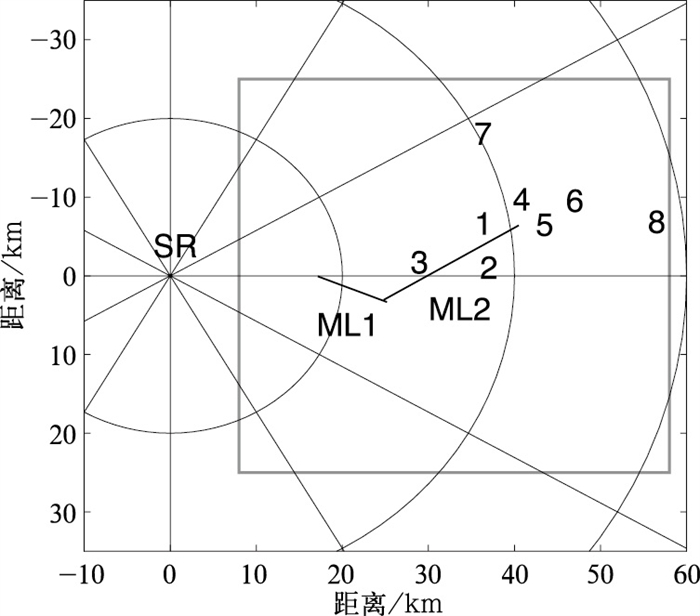图 1 雷达、链路、雨量计的位置分布 “SR”表示S波段天气雷达，数字1~8代表 8个雨量计，实线“ML1”和“ML2”表示2条微波链路; 方框代表包含链路和雨量计在内的50 km×50 km的正方形校准区域; 距离圈为S波段天气雷达的20 km等距离圈 Fig. 1 Positions of the radar, microwave links and rain gauges The S-band weather radar is indicated by "SR" and the eight rain gauges are indicated by the thick numbers; the two microwave links are indicated by solid lines "ML1" and "ML2"; the square box represents the 50 km×50 km square area of calibration; the circles correspond to S-band radar ranges of 20, 40 and 60 km, respectively

S波段天气雷达发射频率为2.85 GHz，探测范围为460 km，峰值功率为650 kW，天线高度为138 m，距离库长1000 m，波束宽度为0.99°，进行周期性的体积扫描，每6分钟完成11个仰角层的探测。试验中使用S波段雷达最低仰角(0.5°)层的数据进行降水反演，需首先进行地物消除和滤波处理，以减少地杂波和随机噪声的影响。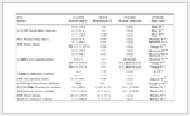表 1 雨量计与天气雷达和微波链路的相对位置 Table 1 The positions of the rain gauges relative to weather radar and microwave link
3 结果与分析

3.1 降水过程Ⅰ：2016年6月1日降水过程

2016年6月1日，南京地区经历了一次暴雨过程，降水从08:06—13:00(以下简称降水过程Ⅰ)持续约5 h，将S波段天气雷达进行一次体积扫描的时间(6 min)作为一个时次，此次降水对应50个时次。第9时次(08:54)的雷达回波强度如图 2所示。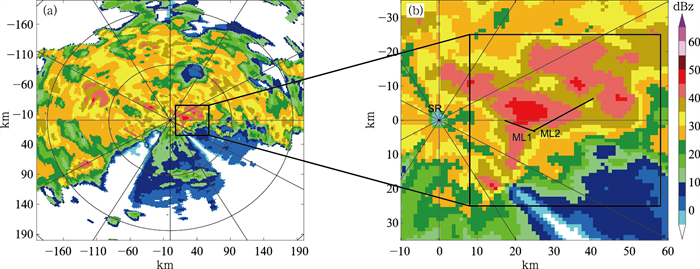图 2 2016年6月1日08:54(a)S波段雷达回波强度(仰角0.5°), (b)图 2a中试验区域的放大 粗实线表示微波链路ML1和ML2 Fig. 2 (a) Reflectivity PPI at 0.5° elevation of the S-band radar, (b) the enlarged view of the experimental area in Fig. 2a at 08:54 BT 1 June 2016 Thick solid lines represent microwave links ML1 and ML2, respectively

(1) 校准因子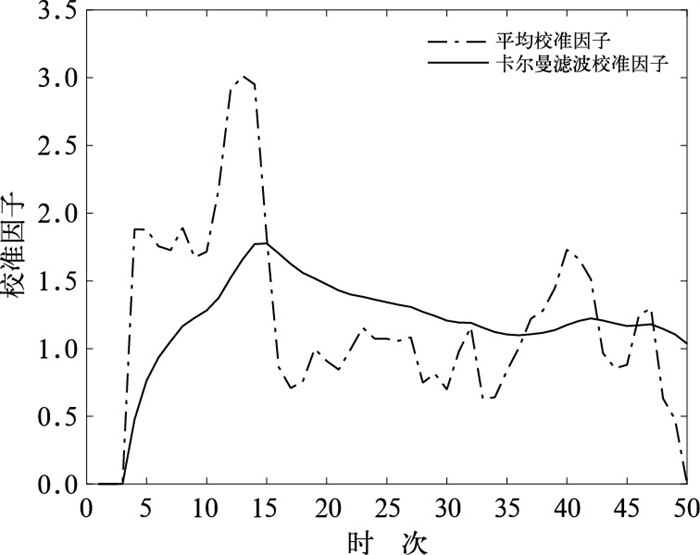图 3 2016年6月1日08:06—13:00平均校准法和卡尔曼滤波校准法计算的校准因子 Fig. 3 The calibration factors calculated by the mean calibration method and the Kalman filter calibration method during 08:06-13:00 BT 1 June 2016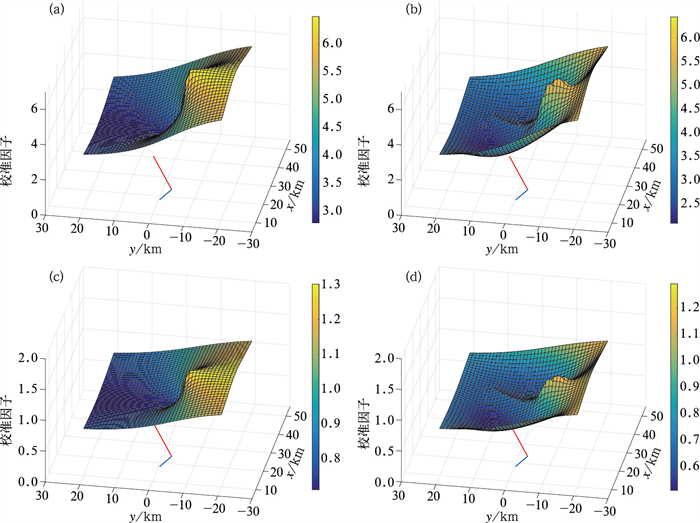图 4 2016年6月1日08:06—13:00(a, c)克里金校准法以及(b, d)变分校准法在(a, b)第13时次(09:18)和(c, d)第28时次(10:48)得到的校准因子分布 红、蓝实线代表 2条微波链路，下同 Fig. 4 Distribution of the calibration factors calculated by (a, c) the Kriging calibration method, (b, d) the variational calibration method at (a, b) the 13th time point (09:18 BT) and (c, d) the 28th time point (10:48 BT) during 08:06-13:00 BT 1 June 2016 The red and blue lines represent the two microwave links, the same below

(2) 校准结果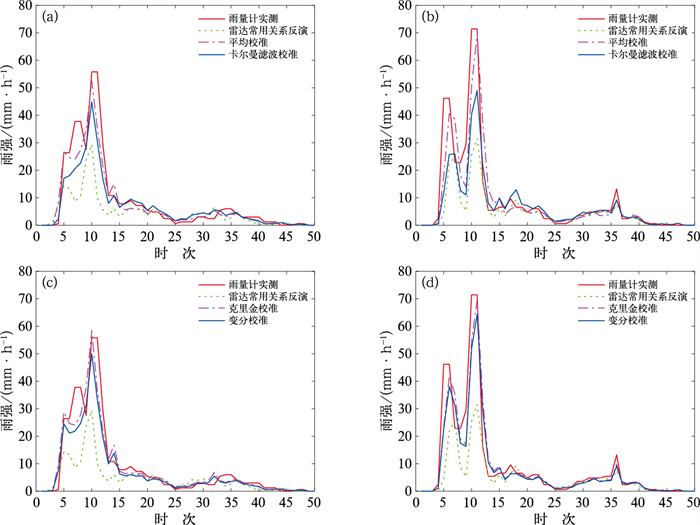图 5 2016年6月1日08:06—13:00(a, b)平均校准法和卡尔曼校准法以及(c, d)克里金校准法和变分校准法在(a, c)雨量计1位置处和(b, d)雨量计5位置处的校准结果 Fig. 5 Calibration results of (a, b) the mean calibration method and the Kalman filter calibration method, (c, d) the Kriging calibration method and the variational calibration method at the position of (a, c) raingauge 1 and (b, d) raingauge 5 during 08:06-13:00 BT 1 June 2016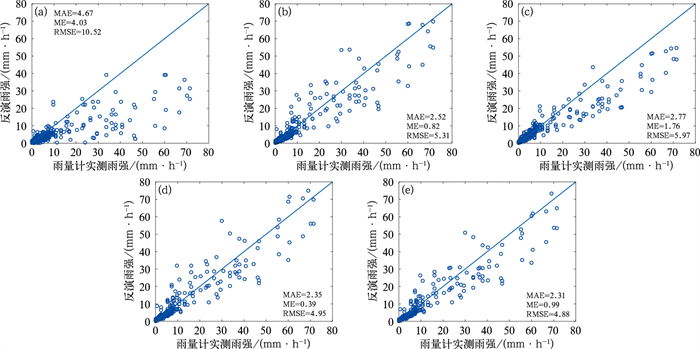图 6 2016年6月1日08:06—13:00雨量计实测雨强与(a)雷达常用降水估计关系反演雨强，(b)平均校准，(c)卡尔曼滤波校准，(d)克里金校准，(e)变分校准后的雷达反演雨强散点图 Fig. 6 Scatter plots of the rain rates measured by rain gauges during 08:06-13:00 BT 1 June 2016 and (a) the radar retrieved rain rates using the default precipitation estimator, calibrated by (b) the mean calibration method, (c) the Kalman filter calibration method, (d) the Kriging calibration method and (e) the variational calibration method

3.2 降水过程Ⅱ：2016年6月12日降水过程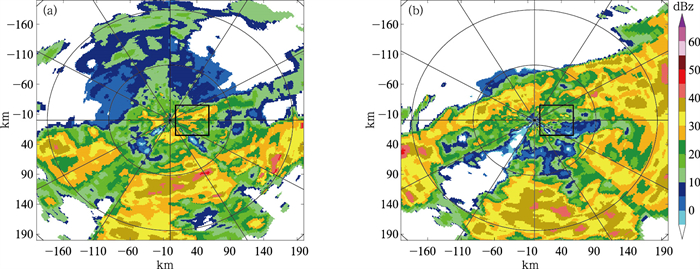图 7 2016年6月12日(a)00:36，(b)06:00的S波段雷达回波强度(仰角0.5°) Fig. 7 Reflectivity PPIs at 0.5° elevation of the S-band radar at (a) 00:36 BT and (b) 06:00 BT 12 June 2016

(1) 校准因子计算结果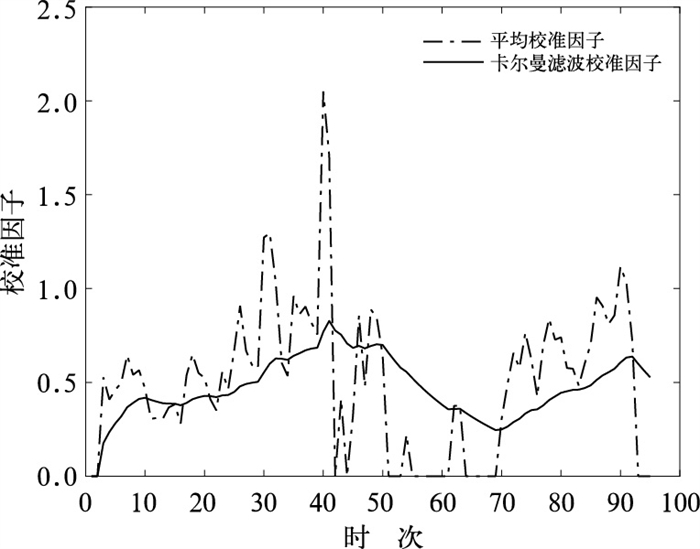图 8 2016年6月12日00:06—09:30平均校准因子和卡尔曼滤波校准因子分布 Fig. 8 Distributions of the mean calibration factors and the Kalman filter calibration factors during 00:06-09:30 BT 12 June 2016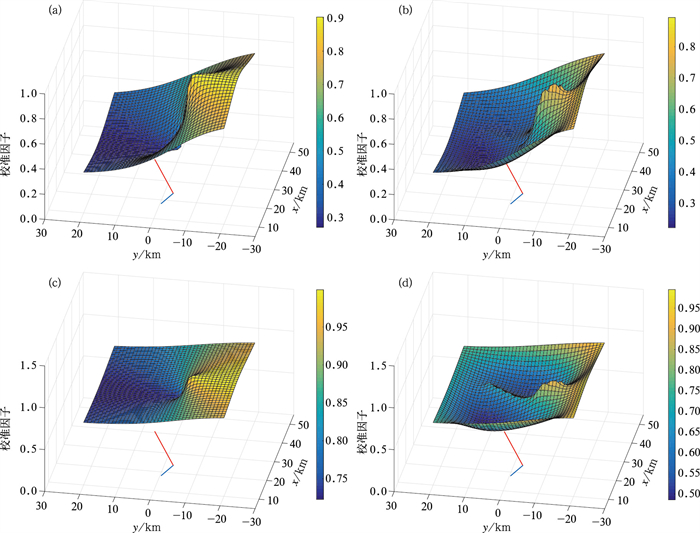图 9 2016年6月12日00:06—09:30(a, c)克里金校准法以及(b, d)变分校准法在(a, b)第12时次(01:12)和(c, d)第80时次(08:00)得到的校准因子分布 Fig. 9 Distributions of (a, c) the Kriging calibration factors, (b, d) the variational calibration factors at (a, b) the 12th time point (01:12 BT) and (c, d) the 80th time point (08:00 BT) during 00:06-09:30 BT 12 June 2016

(2) 雨强校准结果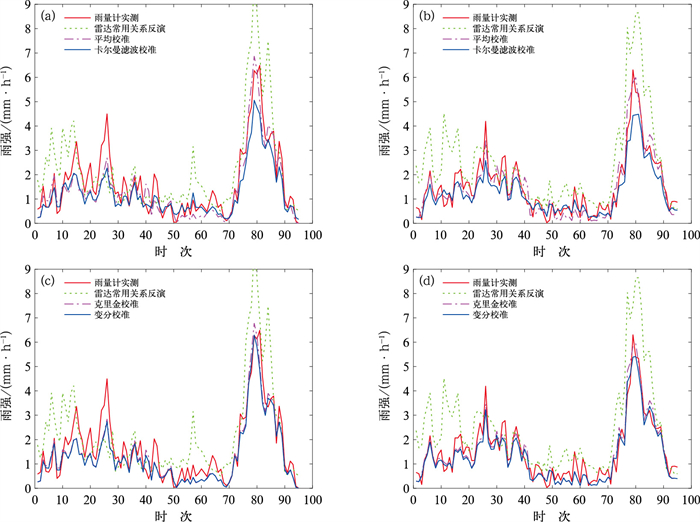图 10 同图 5，但为2016年6月12日00:06—09:30 Fig. 10 Same as Fig. 5, but during 00:06-09:30 BT 12 June 2016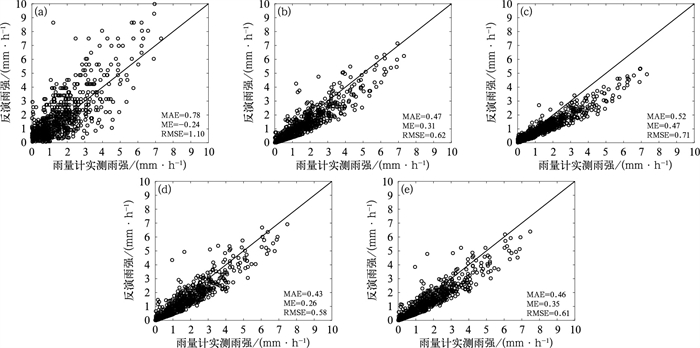图 11 同图 6，但为2016年6月12日00:06—09:30 Fig. 11 Same as Fig. 6, but during 00:06-09:30 BT 12 June 2016
3.3 两次降水过程综合分析表 2 四种方法的误差统计 Table 2 Statistics of the errors of the four methods

4 结论

(1) 通过平均校准法、卡尔曼滤波校准法、克里金校准法和变分校准法，利用2条微波链路，对2500 km2区域的两次降水过程的雷达降水场进行校准，均取得了较好的校准效果，改善了降水过程Ⅰ对流性强降水的低估问题和过程Ⅱ对流性弱降水的高估问题。即使是相对简单的平均校准法，也起到了一定的效果。与校准前相比，校准后的雷达降水场与雨量计实测雨强的一致性得到提升，统计误差得到明显改善，对ME、RMSE和MAE的优化率分别达到75.26%、52.71%和44.57%。

(2) 总体上，克里金校准方法的效果相对较好，变分校准方法和平均校准方法的效果优于卡尔曼滤波校准方法。对统计误差MAE和ME的改善效果最好的均为克里金校准法，对RMSE改善效果最好的是变分校准法。

(3) 平均校准法和卡尔曼滤波校准法得到的校准因子仅随时间变化，不随空间位置变化，而克里金校准法和变分校准法得到的是校准因子场，随时间和空间不同而变化。